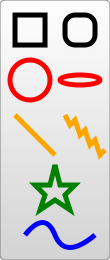/SVG

# Basic Shapes

There are several basic shapes used for most SVG drawing. The purpose of these shapes is fairly obvious from their names. Some of the attributes that determine their position and size are given, but an element reference would probably contain more accurate and complete descriptions along with other properties that won't be covered in here. However, since they're used in most SVG documents, it's necessary to give them some sort of introduction.

## Basic shapes

To insert a shape, you create an element in the document. Different elements correspond to different shapes and take different attributes to describe the size and position of those shapes. Some are slightly redundant in that they can be created by other shapes, but they're all there for your convenience and to keep your SVG documents as short and as readable as possible. All the basic shapes are shown in the image to the right. The code to generate that looks something like:```<?xml version="1.0" standalone="no"?>
<svg width="200" height="250" version="1.1" xmlns="http://www.w3.org/2000/svg">

<rect x="10" y="10" width="30" height="30" stroke="black" fill="transparent" stroke-width="5"/>
<rect x="60" y="10" rx="10" ry="10" width="30" height="30" stroke="black" fill="transparent" stroke-width="5"/>

<circle cx="25" cy="75" r="20" stroke="red" fill="transparent" stroke-width="5"/>
<ellipse cx="75" cy="75" rx="20" ry="5" stroke="red" fill="transparent" stroke-width="5"/>

<line x1="10" x2="50" y1="110" y2="150" stroke="orange" stroke-width="5"/>
<polyline points="60 110 65 120 70 115 75 130 80 125 85 140 90 135 95 150 100 145"
stroke="orange" fill="transparent" stroke-width="5"/>

<polygon points="50 160 55 180 70 180 60 190 65 205 50 195 35 205 40 190 30 180 45 180"
stroke="green" fill="transparent" stroke-width="5"/>

<path d="M20,230 Q40,205 50,230 T90,230" fill="none" stroke="blue" stroke-width="5"/>
</svg>```
Note: The `stroke`, `stroke-width` and `fill` attributes are explained later in the tutorial.

### Rectangles

The rect element does exactly what you would expect and draws a rectangle on the screen. There are really only 6 basic attributes that control the position and shape of the rectangle on screen here. The image shown earlier shows two rect elements, which I admit is a bit redundant. The one on the right has its rx and ry attributes set, giving it rounded corners. If they're not set, they default to 0.

```<rect x="10" y="10" width="30" height="30"/>
<rect x="60" y="10" rx="10" ry="10" width="30" height="30"/>```
x
The x position of the top left corner of the rectangle.
y
The y position of the top left corner of the rectangle.
width
The width of the rectangle
height
The height of the rectangle
rx
The x radius of the corners of the rectangle
ry
The y radius of the corners of the rectangle

### Circle

As you would have guessed, the circle element draws a circle on the screen. There are really only 3 attributes that are applicable here.

`<circle cx="25" cy="75" r="20"/>`
r
The radius of the circle.
cx
The x position of the center of the circle.
cy
The y position of the center of the circle.

### Ellipse

Ellipses are actually just a more general form of the circle element, where you can scale the x and y radius (commonly called the semimajor and semiminor axis by math people) of the circle separately.

`<ellipse cx="75" cy="75" rx="20" ry="5"/>`
rx
The x radius of the ellipse.
ry
The y radius of the ellipse.
cx
The x position of the center of the ellipse.
cy
The y position of the center of the ellipse.

### Line

Lines are again, just straight lines. They take as attributes two points which specify the start and end point of the line.

`<line x1="10" x2="50" y1="110" y2="150"/>`
x1
The x position of point 1.
y1
The y position of point 1.
x2
The x position of point 2.
y2
The y position of point 2.

### Polyline

Polylines are groups of connected straight lines. Since that list can get quite long, all the points are included in one attribute:

`<polyline points="60 110, 65 120, 70 115, 75 130, 80 125, 85 140, 90 135, 95 150, 100 145"/>`
points
A list of points, each number separated by a space, comma, EOL, or a line feed character. Each point must contain two numbers, an x coordinate and a y coordinate. So the list (0,0), (1,1) and (2,2) could be written: "0 0, 1 1, 2 2".

### Polygon

Polygons are a lot like polylines in that they're composed of straight line segments connecting a list of points. For polygons though, the path automatically returns to the first point for you at the end, creating a closed shape. Note that a rectangle is a type of polygon, so a polygon can be used to create a `<rect/>` element in cases where you need a little more flexibility.

`<polygon points="50 160, 55 180, 70 180, 60 190, 65 205, 50 195, 35 205, 40 190, 30 180, 45 180"/>`
points
A list of points, each number separated by a space, comma, EOL, or a line feed character. Each point must contain two numbers, an x coordinate and a y coordinate. So the list (0,0), (1,1) and (2,2) could be written: "0 0, 1 1, 2 2". The drawing then closes the path, so a final straight line would be drawn from (2,2) to (0,0).

### Path

Path is probably the most general shape that can be used in SVG. Using a path element you can draw rectangles (with or without rounded corners), circles, ellipses, polylines, and polygons. Basically any of the other types of shapes, bezier curves, quadratic curves, and many more. For that reason, paths alone will be the next section in this tutorial, but for now I will just point out that there is a single attribute used to control its shape.

`<path d="M20,230 Q40,205 50,230 T90,230" fill="none" stroke="blue" stroke-width="5"/>`
d
A list of points and other information about how to draw the path. See the Paths section for more information.

© 2005–2018 Mozilla Developer Network and individual contributors.
Licensed under the Creative Commons Attribution-ShareAlike License v2.5 or later.
https://developer.mozilla.org/en-US/docs/Web/SVG/Tutorial/Basic_Shapes